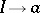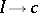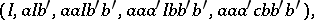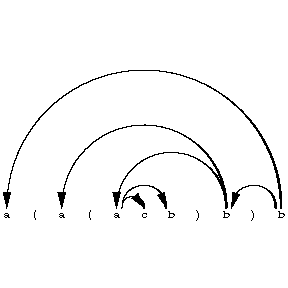# Grammar, dominating

(diff) ← Older revision | Latest revision (diff) | Newer revision → (diff)

A type of formal grammar (cf. Grammar, formal) serving to generate strings together with derivation trees (cf. Syntactic structure). Formally, a dominating grammar can be defined as a context-free grammar (cf. Grammar, context-free) in which one of the occurrences of symbols on the right-hand side carries a special mark, except for rules of the typewhereis the initial symbol andis a terminal symbol. The right-hand side of each such rule must contain not fewer than two symbol occurrences. The context-sensitive system corresponding to the derivation in such a grammar (cf. Grammar, context-sensitive) becomes hierarchic if the components "originating" from the marked occurrences of symbols on the right-hand side of the rules are considered to be the terminal components. Each string of the language generated by the grammar is represented by a "derivation" tree, connected with this hierarchic context-free system (it is usually not unique). The diagram shows one such tree, which represents the stringin the grammar with rules,,(is the initial symbol, while the prime serves as the mark); this tree corresponds to the derivationand the parentheses serve to separate non-trivial components.Figure: g044800a

The most important partial class of dominating grammars are the so-called simple dominating grammars, in which only terminal symbols appear on the right-hand sides of the rules. Thus, the grammar of the example shown above is simple. For any simple dominating grammar there exists a natural numbersuch that in each "derivation" tree representing some string in this grammar not more thanarcs issue out of any vertex. Conversely, each dominating grammar with this property has an equivalent simple dominating grammar such that, for any string, the sets of "derivation" trees defined for it by the two grammars are identical.

A simple dominating grammar is also called a dependency grammar.

How to Cite This Entry:
Grammar, dominating. Encyclopedia of Mathematics. URL: http://encyclopediaofmath.org/index.php?title=Grammar,_dominating&oldid=18932
This article was adapted from an original article by A.V. Gladkii (originator), which appeared in Encyclopedia of Mathematics - ISBN 1402006098. See original article